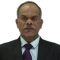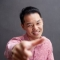How to create a complex number in Python?Published on 06-Feb-2018 06:59:02
Complex number is made up of real and imaginary parts. Real part is a float number, and imaginary part is any float number multiplied by square root of -1 which is defined as j.>>> no=5+6j >>> no.real 5.0 >>> no.imag 6.0 >>> type(no) <class 'complex'>The resulting object is of complex ... Read More

How do we evaluate a string and return an object in Python?Published on 06-Feb-2018 07:02:16
The built in eval() function requires a string argument. However Python interpreter treats the string as a Python expression and evaluates if it is valid and then returns the type object resulting from expression.String containing arithmetic expression>>> x=eval('2+2') >>> type(x) <class 'int'> >>> x 4String evaluating to list/tuple>>> x=eval('tuple([1, 2, ... Read More

How can I convert Python strings into tuple?Published on 06-Feb-2018 07:04:22
Python’s built-in function tuple() converts any sequence object to tuple. If it is a string, each character is treated as a string and inserted in tuple separated by commas.>>> string="Tutorialspoint" >>> tuple(string) ('T', 'u', 't', 'o', 'r', 'i', 'a', 'l', 's', 'p', 'o', 'i', 'n', 't')Any non-sequence object as argument ... Read More

What does the Double Star operator mean in Python?Published on 06-Feb-2018 07:07:38
For numeric data types double asterisk (**) is defined as exponentiation operator>>> a=10; b=2 >>> a**b 100 >>> a=1.5; b=2.5 >>> a**b 2.7556759606310752 >>> a=3+2j >>> b=3+5j >>> a**b (-0.7851059645317211+2.350232331971346j)In a function definition, argument with double asterisks as prefix helps in sending multiple keyword arguments to it from calling environment>>> ... Read More

How to get the size of a list in Python?Published on 06-Feb-2018 07:09:37
Object of any Python sequence data type including list uses a built-in function len() which returns its size i.e. number of elements in it.>>> L1=[1, 2, 3] >>> len(L1) 3Built-in list class has a special method called __len__() which also returns size of list.>>> L1=[1, 2, 3] >>> L1.__len__() 3Read More

What does the Star operator mean in Python?Published on 06-Feb-2018 07:12:59
The asterisk (star) operator is used in Python with more than one meaning attached to it.For numeric data types, * is used as multiplication operator>>> a=10;b=20 >>> a*b 200 >>> a=1.5; b=2.5; >>> a*b 3.75 >>> a=2+3j; b=3+2j >>> a*b 13jFor sequences such as string, list and tuple,  * is ... Read More

How do we return multiple values in Python?Published on 06-Feb-2018 07:17:45
It is possible to return multiple values from a function in the form of tuple, list, dictionary or an object of a user defined classReturn as tuple>>> def function():       a=10; b=10       return a, b >>> x=function() >>> type(x) <class 'tuple'> >>> x (10, ... Read More

converting array to list in javaPublished on 06-Feb-2018 07:04:05
Following program is converting an Integer[] to List<Integer> −Exampleimport java.util.Arrays; import java.util.List; public class Tester {    public static void main(String[] args) {       Integer[] numbers = {1, 2, 3, 4, 5};       List<Integer> list = Arrays.asList(numbers);       System.out.println(list);    } }Output[1, 2, 3, ... Read More

How to create a dictionary with list comprehension in Python?Published on 06-Feb-2018 07:19:22
The zip() function which is an in-built function, provides a list  of tuples containing elements at same indices from two lists. If two lists are keys and values respectively,  this zip object can be used to constructed dictionary object using another built-in function dict()>>> L1=['a', 'b', 'c', 'd'] >>> L2=[1, ... Read More

What does ** (double star) and * (star) do for parameters in Python?Published on 06-Feb-2018 07:22:16
In Python function, an argument with single asterisk (star) prefixed to it helps in receiving variable number of argument from calling environment>>> def function(*arg):       for i in arg:         print (i) >>> function(1, 2, 3, 4, 5) 1 2 3 4 5Argument with ... Read More